Mesh Deformation Approaches and#8211; A Survey | OMICS International
Journal of Physical Mathematics
All submissions of the EM system will be redirected to Online Manuscript Submission System. Authors are requested to submit articles directly to Online Manuscript Submission System of respective journal.

# Mesh Deformation Approaches – A Survey

Selim MM1* and Koomullil RP2

1Department of Material Science & engineering University of Alabama, USA

2Department of Mechanical engineering, University of Alabama, USA

*Corresponding Author:
Selim MM
Associate Professor
Department of Material Science & engineering,
University of Alabama, USA
Tel: 205-493-1311
E-mail: [email protected]

Received Date: April 08, 2016; Accepted Date: June 22, 2016; Published Date: June 27, 2016

Citation: Selim MM, Koomullil RP (2016) Mesh Deformation Approaches – A Survey. J Phys Math 7:181. doi:10.4172/2090-0902.1000181

Copyright: © 2016 Selim MM, et al. This is an open-access article distributed under the terms of the Creative Commons Attribution License, which permits unrestricted use, distribution, and reproduction in any medium, provided the original author and source are credited.

Visit for more related articles at Journal of Physical Mathematics

#### Abstract

This survey reviews the recent development of mesh deformation methods. During the past two decades a vast number of researches have been concerned with developing an efficient and robust mesh deformation technique. This has been achieved either by proposing a novel approach, improving an existing one, or by combining two existing approaches together resulting in a new hybrid approach. It is important to keep track of the most up to date developments in the field of mesh deformation, in order to allow the researchers to adopt the most efficient and application compatible scheme as well as to propose new methods of improvements. In this survey the mesh deformation techniques have been classified into two main categories, 1) physical analogy based techniques and 2) interpolation based techniques. The most significant techniques under these two classes are reviewed and the aspects of strength and weaknesses are highlighted.

#### Keywords

Mesh deformation; Spring analogy; Elasticity; Laplacian; Radial basis functions; Delaunay graph; Fluid-structure interaction

#### Introduction

The numerical simulation of dynamically updated threedimensional (3D) meshes arises in many engineering applications, such as moving boundary problems , bio-fluid mechanics problems [2,3] free surface flows, and Fluid–Structure Interaction (FSI) problems . FSI are of great importance in many real-life applications, such as industrial processes, aero-elasticity, and bio-mechanics. In such applications, when the flow domain boundary undergoes a motion, the most common approach is to conform the fluid mesh to confine the changing flow domain

This can be achieved either by deleting the old mesh and regenerating a new mesh or by dynamically deform the mesh. For applications require updating the mesh at every time step, regenerating a new mesh consumes high CPU cost and requires special mesh quality controls which makes it impractical approach [5-7]. Moreover, mapping the solution from the old mesh to the new mesh consumes extra CPU cost. Therefore, the mesh deformation option is more practical and flexible.

Various mesh deformation methodologies have been proposed. Some of them are robust with respect to elements overlapping and crossing but very time consuming, while others are computationally efficient but less robust. Since 1980 it has been a challenge to develop an efficient as well as a robust mesh deformation technique. However, various types of simulations have different mesh deformation requirements. The simplest problem is when an object undergoes a translational or rotational motion, followed by an object experiencing both translational and rotational motions, then the most complicated when the problem involves higher frequency components or multiple objects motions. Moreover, handling structured grids is easier than unstructured grids and dealing with small deformations is obviously simpler than large deformations. Also, viscous flows need a special treatment for preserving the quality of the boundary layer mesh, whereas the mesh layers are tightly packed and needs to move rigidly with the boundary surfaces.

Strategies for deforming the fluid mesh conforming to the deformation of solid body can be divided into two basic classes: physical analogy or interpolation. The physical analogy approach describes the fluid mesh deformation according to a physical process that can be modeled using numerical methods. In the interpolation based approaches, an interpolation function is used to transfer prescribed boundary point displacements to the fluid mesh. The most popular approaches of each of these classes are discussed below.

#### Mesh Deformation using Physical Analogy

Techniques lying under this category are based on spring analogy or solutions of partial differential equations. The main drawback of physical analogy methods is that they involve large systems of equations, implying a higher computational cost. Besides, these methods require grid connectivity information which results in more storage requirements and difficulties in parallelization.

Linear spring analogy

One of the popular methods in this class is the tension spring analogy developed by Batina . In this approach, each edge of the mesh is replaced by a tension spring with the spring stiffness is taken as inversely proportional to the edge length. Many researchers have adopted the spring analogy and also used the same assumption for the stiffness [9-11]. In this method, the equilibrium lengths of the springs are set equal to the initial lengths of the edges. By applying Hook’s law to the nodes displacements, the force is written as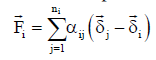(1)

Where αij is the stiffness of the spring between node i and j,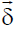is the node displacement and ni is the number of neighbors of node i. For static equilibrium, the force at every node i has to be zero. The iterative equation to be solved is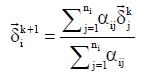(2)

where the known displacements at the boundaries are used as the boundary conditions. After iteratively solving Eq. (2), the nodes coordinates are updated by adding the final displacement to them. Blom  analyzed the stiffness of the springs of a one-dimensional linear spring system. He proved that by setting the stiffness equal to the inverse of the edge length the nodes are prevented from colliding when they are placed on a line and move along this line. In other words, this stiffness choice prevents the cells from colliding if they are placed on the same axis and moving across this axis. However, for triangular grids it is possible for the triangle edges to rotate and cross each other, see Figure 1. In order to prevent this, Farhat proposed the use of torsional springs that are placed in the corner between adjacent edges . An alternative solution is to divide the edge stiffness by the angle formed by the other two edges in the triangle. However, this will result in a non-linear system of equations, since αij will become a function of the displacement. This approach usually referred to as the semi-torsional spring approach.

Modified spring analogies

In order to prevent the element inversion problem associated with the linear spring analogy multiple modifications have been proposed. The most influencing modifications are discussed in this section. These modifications are the torsional spring , the semi-torsional spring , the ball-vertex , and the Ortho-Semi-Torsional (OST) spring approach .

Torsional spring method: To prevent element inversion on a 2D triangular mesh, three torsional springs where attached, one at each vertex of the triangle and their stiffness coefficients were depended on the angle θ of the corresponding vertex in the triangle. The stiffness of the torsional spring is given by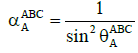(3)

where the subscript A specifies the vertex on which the calculation applies, and the superscript ABC specifies the triangle which the vertex A belongs to.

This method was extended to 3D meshes by Degand and Farhat . They constructed 12 triangles within each tetrahedron, where three triangles are constructed for each vertex. Consider the tetrahedron ABCD, to prevent the vertex D from penetrating through its opposite face ABC, three triangles can be constructed by projecting the vertex D on each of the edges forming the face ABC. The first triangle can be constructed as follow, consider the projection of the vertex D on the edge AB as node X, then the first triangle is DXC, as shown in Figure 2. Similarly, consider the projection of the vertex D on the edges AC and BC as node Y and node Z respectively. Then, the other two triangles are DYB and DZA respectively.

The stiffness coefficients are then calculated using Eq. (3). For

points X, Y, and Z, the displacement is calculated by interpolating the displacements of the two vertices of the corresponding edge. Finally, the local stiffness matrix for the tetrahedron is obtained by assembling all matrices associated with the twelve inserted triangles.

Burg generalized the extension of the method for 3D meshes in order to be applicable for higher order elements, such as quadrilateral .

Ball-vertex spring method: The concept of this method is to modify the original linear spring method by introducing additional linear springs. These additional springs resist the motion of a vertex towards its opposite faces, shown in Figure 3. Point i position is computed as the normal projection of the vertex D on the face ABC. The displacement at i can be calculated by the interpolation of the three vertices of the corresponding face. The stiffness of the added spring is,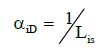(4)

Where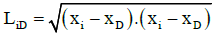(5)

Semi-torsional spring method: This method considers the same springs of the original linear spring method, but with different stiffness calculation approach. Here, the stiffness coefficient of each linear spring is equal to the torsional stiffness coefficient of element, a semitorsional stiffness coefficient of an edge AB is,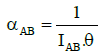(6)

Where lAB is the length of the edge AB and θ is the edge facing angle. This semi-torsional 2D model is not directly applicable to 3D problems. Zeing and Ethier  extended this method for 3D applications. They suggested defining the spring stiffness as the sum of its linear and semitorsional stiffness, as follows,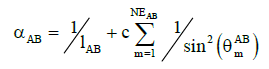(7)

where NEAB is the number of elements sharing edge AB,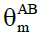is the edge facing angle on the mth element sharing the edge AB, and c is a coefficient which is related to the dimension of the stiffness. Figure 4 shows the facing angle of an edge on a tetrahedron.

Thus, for edge AD the following system of equations results,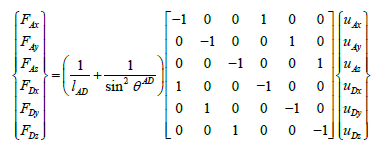(8)

This method requires the calculation of 6 torsional stiffness coefficients corresponding to the 6 angles created by four tetrahedral faces. Hence, this method is more efficient than the torsional spring method, which requires 12 triangles to be created for each tetrahedron. In 2D problems the calculations to form the fictitious stiffness matrix for the torsional spring analogy model involve 72 additions and 117 multiplications per element, while for the semi-torsional spring analogy model these operations are reduced to 18 additions and 20 multiplications per element .

Ortho-semi-torsional spring method: In this method four additional springs, within each tetrahedron element, have been considered in addition to the springs of the original spring method. These four springs are connecting each vertex with its projection on its opposite face, as shown in Figure 5. The stiffness coefficients of these imaginary springs were assumed to be inversely proportional to their lengths. These additional springs are used only to alter the original springs’ stiffness coefficients, and they are discarded in the final formulation. Each additional stiffness coefficient is divided into three parts, one for each neighboring edge, as follow,

Figure 5: Additional projected spring from each node toward its opposite face .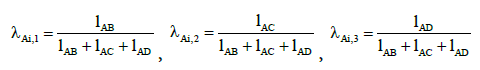(9)

Then each of the neighboring edges stiffness coefficients are calculated as,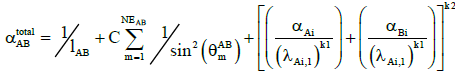(10)

Where k1 is a coefficient is related to the closeness of the projection Ai to the neighboring edges and k2 is a coefficient affects the contribution of the additional linear spring stiffness coefficients to the total stiffness of the edge. This method combines the simplicity of the semi-torsional spring method and the robustness of the torsional spring method. In summary, Markou  conducted several tests in order to analyze each of these four schemes. They concluded that the ball-vertex and semi-torsional spring analogy methods could be an appropriate choice for large problems with relatively small deformations. On the other hand, for large boundary surfaces deformations, then the torsional spring analogy is a better choice. However, for both cases, the OST spring analogy method appeared to be ensuring robustness and computational efficiency.

#### Linear Elasticity

In this method, mesh deformation is accomplished by solving the linear elasticity equations for the mesh point displacements throughout the field. Since the elasticity equations contain material properties, the modulus of elasticity (E) and Poisson’s ratio ( u ), these properties are related to the mesh characteristics. One common approach is to set u as a constant, within the valid physical range from 0 to 1/2, and E either to be calculated as the inverse of the distance between the interior node and the nearest boundary surface or to be set inversely proportional to the cell volume [19-22]. This turned out to be very beneficial for avoiding invalid mesh cells especially near to the boundaries. An alternative approach is to use a constant E and manipulate the υ so that the term 1⁄((1-2υ)) is equal to the aspect ratio of the cell [23,24] or to use constant υ and set E equal to the aspect ratio of the element . This increases the stiffness in regions with high aspect ratio cells leading to more rigid motion near boundary surfaces. Another rarely used option is to set E equal to the element condition number which according to [26,27] should results in the same effect of the previous approach.

Yang and Mavriplis  implemented an adjoint-based optimization procedure for producing a more optimal distribution of E. In this study, an objective function, that was selected to be proportional to the cell volume, was minimized by varying E in each cell. Even though the optimization resulted in avoiding invalid elements generation for highly stretched mixed element meshes, its solution consider to be expensive in terms of CPU time.

Hsu proposed to perform the deformation through two consecutive steps . The first step is performed with uniform modulus of elasticity (E=1) and the second one with varying modulus of elasticity. The element strain energy density output from the first analysis is used to compute the modulus of elasticity for the second analysis.

Most of the studies concerned with the linear elasticity mesh deformation method used the Finite Element method for discretizing the linear elasticity equations and then solved the resulted linear system using GMRES method [19,25,29-31].

#### Laplacian and Modified Laplacian

Using the Laplace smoothing equations for mesh deformation proved to be an efficient technique. The idea behind using the Laplace equations for mesh deformation is that the solution of the Laplace equations satisfies the minimum/maximum principle. In other words, this means that the values of the interior displacements are bounded by the values on the boundary surfaces. This ensures that the interior nodes will not cross the boundaries. The traditional Laplacian method is to consider the Laplace smoothing equation

where u is the mesh deformation velocity such that, ∇.(γq∇u)=0

∇.(∇u)=0 (11)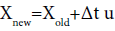(12)

The modified Laplacian includes the factor g raised to some exponentq,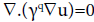(13)

Where γis the diffusion coefficient, If q=0, then the traditional Laplacian is recovered. The selection of variable should depend on the specific mesh motion problem. Jasak and Tuković  investigated several possibilities to set the γ value based on distance (linear, quadratic, and exponential) from some boundary or mesh characteristics (orthogonality and skewness). These methods all have their merits and shortcomings. Lohner  varied the diffusion coefficient with the distance from the viscous surfaces, and Crumpton and Giles  used diffusion coefficient inversely proportional to the cell volume.

One disadvantage, that limits the use of traditional Laplacian method, is that the three components of the mesh deformation are solved independently of each other. For example, if the boundary surface is moved only along x-direction, the interior mesh points will be moved only along coordinate x .

Choosing an appropriate exponent to the modified Laplacian, for single frequency deformations, would highly improve the capability of handling extreme deformations. Therefore, the modified Laplacian yields excellent results in cases of rigid translations and rotations. However, in practical problems, the mesh deformation has multiple frequencies which make the use of optimal exponent impractical and leads to invalid mesh generation .

#### Mesh Deformation using Interpolation Analogy

In general, these schemes do not require connectivity information. Therefore, these algorithms can be applied to arbitrary mesh types that contain general polyhedral elements or hanging nodes . Interpolation based schemes attain higher computational efficiencies and less memory requirements compared to physical schemes. However, any interpolation process is associated with some sort of error margin.

Transfinite interpolation

Most structured grid regeneration and deformation techniques are based on transfinite interpolation (TFI) . In this method, an interior fluid node motion is assumed to be equal to the motion of the moving boundary times a scale factor. This scale factor depends on the distances from the node to the moving and the fixed surfaces [38,39]. This method is very computationally efficient but suffers from robustness issues. Obviously, TFI method does not have any mechanism for preventing element crossing and overlapping, thus the method is not suitable for handling unstructured meshes especially when large deformations take place.

Algebraic damping method

The method, proposed by Zhao and Forhad , works by assigning a boundary node (xbi) for each interior node (xi) that needs to be deformed, then the deformation of this interior node is calculated as the product of a distance function and the displacement of the associated boundary node as follows: || xi-xbi ||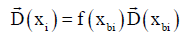(14)

where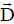is the displacement vector and f is the distance function. The boundary node that has the shortest distance to the interior node is selected as the associated node (xbi). A generic distance function was chosen so it tends to 1 when || xi-xbi || tends to 0 and the function tends to 0 when || xi-xbi || tends to the max || xi-xbi ||for all interior nodes. This results in having a very rigid mesh in areas near to the boundary walls and far away from the boundary wall while having a very elastic mesh in between. In order to improve the robustness of this method for large mesh deformations, a smoothing procedure was also incorporated to eliminate highly skewed or overlapping cells.

Inverse distance weighting method

This method has been originally used for the generation of contour maps in geography . In this method, the interpolated displacement value is an average of the known values at the boundary nodes weighted by the inverse of the distance to the interior fluid node. Thus, the displacement at any interior node xi can be calculated by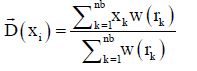(15)

with weighting function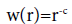(16)

where nb is the total number of boundary nodes, rk=|| xi-xk ||, and c is a power parameter which is usually set to a value of 2 in order to influence the distance-decay effect.

The IDW technique has the capability of treating boundary rotations separately. Therefore, the IDW technique can be used as a tool to improve the mesh orthogonality near the boundary surfaces. To illustrate this property, a single-block structured C-type inviscid mesh was tested by Witteveen . Different power parameters were considered. The results showed that for power parameter c=1 the mesh orthogonality tends to be worst, however, for increasing c the mesh quality improves spectacularly. The mesh quality measure shows a perfect value of 90o over more than 95% of the airfoil surface for c=3. Further increasing of c does not have noticeable effect on mesh quality. However, the effect of this optimization on the global mesh quality was not reported in this study.

In the research conducted by Witteveen , the 3D AGARD aeroelastic wing case has been tested as a full fluid-structure interaction problem. The results for IDW and RBF mesh deformation were compared. The results reported a reduction of computational costs for IDW mesh deformation with respect to the RBF method of a factor 20. Furthermore, by neglecting mesh rotation and consider only mesh translation the reduction of computational costs reduce the CPU time to a factor 50 with respect to RBF mesh deformation. However, the RBF technique produced 4% higher mesh quality than the IDW. It should be noted here that the study did not declare whether the used RBF approach is the most straightforward approach or the improved approach.

The main advantages of this approach can be summarized as, 1) it does not involve the solution of a matrix system of equations, and 2) it treats boundary node displacements and rotations separately.

The radial basis function interpolation method, such as the method developed by Boer , is one of the promising interpolation schemes. RBF’s have become a well-established tool to interpolate scattered data. RBF can also be used as an interpolation function to transfer the displacements known at the boundaries of the structural mesh to the fluid mesh. This scheme produces high-quality meshes with reasonable orthogonality preservation near deforming boundaries. Other advantages of RBF includes: 1) Avoid the need for mesh connectivity information, 2) the system of equations which needs to be solved is linear, and 3) the size of the linear system of equation is proportional to the number of boundary nodes, not all fluid nodes. Moreover, many studies have investigated different techniques for improving RBF’s interpolation based mesh deformation. The most influencing study was made by Rendall and Allen  They proposed the use of data reduction algorithm along with RBF interpolation. This technique will be discussed later in details. Another study, which builds up on the previous technique, is the work made by Sheng and Allen , in which they put forward specific criteria for selecting the nodes involved in the interpolation.

Using RBF, the interpolation function, S, describing the displacement in the whole domain can be approximated by a sum of basis functions as,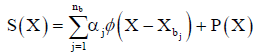(17)

where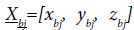are the boundary nodes in which the deformations are known and these are called the centers for RBF, P is a polynomial, nb is the number of boundary nodes, and φ is the selected basis function with respect to the Euclidean distance || x ||. The coefficients αj and the polynomial P are determined by the interpolation conditions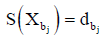(18)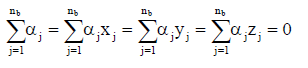(19)

The values for the coefficients αj and the linear polynomial coefficient can be obtained by solving the system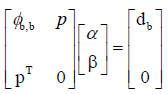(20)

α is a vector containing the coefficients αj, β is a vector containing the coefficients of the linear polynomial P, φb,b is an nb x nb matrix containing the evaluation of the basis function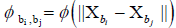,and p is an nb x 4 matrix with row j given by bj bj bj [1 x y z ] [43,46]. In this study, the polynomial P was omitted, since it was concluded in previous studies that it does not have a large influence on the quality of the deformed mesh . In this case, the system of Eq. (20) will be simplified as the following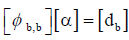(21)

RBF interpolation method produces high-quality meshes with good orthogonality preservation near deforming boundaries. On the other hand, in its most straightforward implementation, it is too costly to use for large 3-D problems. A direct solution of such systems require O(nb 3) operations and O(nb 2) memory usage which becomes prohibitive for more than a few thousand data points. Great progress has been made in recent years towards alleviating this computational burden.

An approximation algorithm for RBF mesh deformation has been sugges ted by Rendall and Allen . In this algorithm, the RBF is applied using a coarsened subset of the surface mesh. Displacements of the omitted surface nodes are calculated using the interpolation method and the error is calculated as the difference between the interpolated values and the actual displacement. A greedy algorithm is used to add points that have the largest error, as illustrated in Figure 6 . Rendall and Allen reported that this algorithm improves the performance of the RBF method by approximately two orders of magnitude. The use of the greedy algorithm reduces the cost remarkably without compromising the accuracy. Selim and Koomullil  introduced the concept of solving the RBF system incrementally within the greedy algorithm. Their incremental solver takes advantage of the RBF system similarity at each greedy iteration with the previous iteration. They showed that their incremental solver saves up to 60% of the CPU time over the traditional solvers. Michler  proposed a confinement technique that restricts the mesh deformation to the surrounding region of the moving surface. He achieved this by assigning an auxiliary geometry encompassing the region targeted by the interpolation, instead of using a cut-off function. Moreover, Michler  proposed to choose different centers for different directions instead of using the same set of centers for all displacement directions, which results in reducing the required CPU time.

Figure 6: RBF deformation of a 2D plate into different shapes showing the selected subset of the surface nodes .

#### Control Mesh Methods

Delaunay graph method

The proposed scheme is based on the creation of a Delaunay graph of the original mesh. The Delaunay graph is used as an intermediate map. Only boundary nodes are used to create the graph. Then each interior node is assigned to the Delaunay element that it belongs to. Finally, by deforming the Delaunay graph, which the displacements are already known for, the interior nodes new locations are easily interpolated . Basically, this method includes the following four steps:

1. Generating the Delaunay graph

2. Locating the mesh points in the graph

3. Moving the Delaunay graph according to the specified geometric change

4. Relocating the mesh points in the new graph

Generating the delaunay graph: The Delaunay graph must be generated to cover the whole computational domain. All moving boundary nodes with few stationary boundary nodes must be used for generating the graph. If not all moving boundary nodes are used, the integrity of the grid is not guaranteed. If the stationary boundary surface has a curved shape, more points on the surface must be used. Then the Delaunay graph is generated using the Delaunay criterion [51,52]. Locating the mesh points in the Delaunay graph

Any node within the graph must belong to one of the triangles or tetrahedrons elements in the graph, since the graph covers the whole solution domain. In order to locate the mesh nodes in the graph, the relative area/volume coefficients to define the points for 2D/3D meshes are used. For 2D, assume that the node P is to be located within the Delaunay element ABC. By connecting the node P with each node of the Delaunay graph element ABC, three sub-triangles will be created. The area coefficients can be calculated as the area of the sub-triangle divided by the area of the element ABC. Similarly, for 3D the volume coefficients can be calculated as the volume of the sub-tetrahedron divided by the volume of the tetrahedron ABCD. Obviously, the summation of all coefficients must equals to 1. However, if and only if all the signs of the above coefficients are positive or zero, the point is within the graph element. Otherwise the point is classified outside the element.

An efficient walk-through algorithm [53,54] was adopted for locating the nodes within the Delaunay graph. The complexity of the walk-through algorithm is of O(n1/d), where n is the total number of the Delaunay elements and d is the spatial dimension.

Moving the delaunay graph: After deforming the mesh, the Delaunay graph might not satisfy the Delaunay rules. This should not cause a problem unless some nodes move across each other, which will results in negative coefficients. In this case, the movement is broken into two smaller steps.

Relocating the mesh points in the graph: After the graph movement, the mesh points can now be located based on the area/ volume coefficients stored for the points with the associated graph element number. This requires keeping the coefficients constants during the deformation.

Test cases: Liu  showed the robustness of the method through a series of test cases including in viscid and viscous flow grids with large deformations. The performance of the method was compared with a standard spring analogy method in the wing-body test case. An order of magnitude improvement in CPU has been achieved, but on the other hand the memory requirements have been increased.

Main disadvantage: Intersections occur occasionally between the Delaunay graph’s elements for complex geometries with large relative movements. Under this condition, the Delaunay graph has to be regenerated and the grid nodes are required to be relocated, which increase the time consumption.

#### RBFs-MSA hybrid method

This method, recently proposed by Yu , combines the benefits of Moving Submesh Approach (MSA) and RBFs interpolation, which is called RBFs-MSA. The MSA method can be considered as an extension to the Delaunay graph interpolation method. The main difference between the two methods that in the MSA the background mesh is not a Delaunay graph anymore, it is a coarse unstructured mesh. This change avoids the elements crossing problem associated with using the Delaunay graph as the background mesh. On the other hand, since the background mesh now is not formed only by boundary nodes, the displacement is not known at all background nodes. Hence, an extra step needs to be performed before transferring the movement from the background mesh to the computational mesh. This step involves interpolating the displacements between the boundary nodes and the non-boundary nodes of the background mesh. Therefore, the authors proposed the use of RBFs interpolation to perform this step. Since the background mesh is a coarse mesh, performing RBFs interpolation will be efficient.

RBFs-MSA hybrid algorithm’s steps:

• Generate the background meshs

• Locate the computational mesh nodes on the background mesh element and compute the area/volume ratio coefficients

• Update the coordinates of the boundary points of the background mesh

• Solve the RBF interpolation system and evaluate the nonboundary points new coordinates of the background mesh

• Update the coordinates of computation mesh points by interpolation using the relative area/ volume ratio coefficients

• Repeat Step 3 to Step 5 until the end of computation

Test cases results: Two 2D test cases and one 3D test case have been presented in . Firstly, a rectangular block rotation 2D test case was analyzed and compared with RBFs interpolation and semi torsional spring scheme. The results showed that the semi torsional spring produces an invalid mesh after 50° rotation. On the other hand, the RBFs-MSA and the RBFs interpolation produce a valid mesh for 90° rotation. However, the RBFs interpolation produced slightly better mesh quality than the RBFs-MSA. Secondly, a double flapping wings 2D test case was analyzed and compared with RBFs interpolation. The RBFs-MSA produced slightly better mesh quality than the RBFs interpolation. However, the RBFs-MSA reduced the CPU cost by about two orders of magnitude in comparison to RBFs interpolation.

Finally, a 3D test case was constructed by artificially bending the ONERA M6 wing. Since the computational mesh in this case has 10,419 boundary nodes, the authors stated that using RBFs interpolation is unpractical. Thus, the RBFs-MSA has been compared to the semitorsional spring method. The comparison was in favor of the RBFs- MSA method. It showed that the RBFs-MSA method produced an acceptable mesh quality while the semi-torsional method produced poor mesh quality. Moreover, the RBFs-MSA was 6.35 times faster than the semi-torsional spring method.

Quaternion based method

A quaternion can be interpreted as a scalar with a direction. It is composed of one real number and three imaginary numbers, on the form,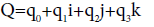(22)

where ii=jj=kk=-1, ij=-ji=k, jk=-kj=i, and ki=-ik=j

Quaternions are widely used to describe rotations in computer graphics, animations, control theories in robotics, attitude controls of spacecraft, etc. Quaternions have several unique properties, such as : Q-1=Q*/ Q Q*

Conjugate of a quaternion, Q*=q0- q1i- q2j- q3k

Magnitude of a quaternion,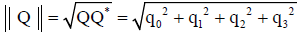Unit quaternion, || Q ||=1

Inverse of quaternion, Q-1=Q*/ Q Q*

For unit quaternion, Q-1=Q*

The nature of the quaternion makes it ideal to carry on rotational information, for which the scalar term could represent the magnitude of the rotation and the last three terms represent the axis of rotation. Moreover, the unique properties those quaternions have make it easier to perform different mathematical operations on them.

Samareh  proposed a technique to use quaternions for mesh deformation. He proposed a three steps approach to translate the known boundary displacements into boundary quaternions and then use these quaternions to deform the mesh. Step 1) is to translate the undeformed nodes, deformed nodes, and the neighboring nodes to the origin. Step 2) is to rotate the undeformed nodes so their normal vectors align with the deformed nodes normal vectors. This step is performed using a quaternion, which is calculated as follow,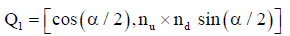(23)

Where αthe angle between the two normal, and nu and nd is are the undeformed and deformed normal, respectively. Step 3) is to rotate the undeformed nodes about the deformed boundary normal vectors in order to minimize the angle between neighboring nodes. Similarly, another quaternion can be used to perform this rotation,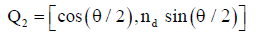(24)

Where θ is the average angle between corresponding neighboring nodes. Step 4) is to compute the total rotation of any node as the composition of Q1 and Q2 as,

Q=Q1. Q2 (25)

And the translation vector T is calculated as,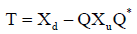(26)

Where Xd and Xu is the deformed and undeformed node position vector, respectively. The translation vectors and quaternions are propagated into the field mesh using the spring analogy. Maruyama  proposed a modification to this method in order to generalize the formulation and obtain the quaternions independently of the coordinate system. They used Laplacian smoothing to propagate the translation vectors and the quaternions into the field. Moreover, they reported that this method is at least one order of magnitude more CPU intensive than other interpolation based mesh deformation methods, such as RBF interpolation method.

Worth mentioning approaches

Recently, a novel interpolation based scheme has been developed by Luke . In this scheme, the deformation of the volume mesh is viewed as a projection of the surface deformation into the volume. Using a tree-code optimization, the algorithm cost is demonstrated to be O(nlog(n)), where n is the total number of nodes in the simulation, with mesh quality that is competitive to radial basis function (RBF) scheme.

McDaniel and Morton  developed a technique that is based on a two-pronged approach where the viscous layers of nodes are deformed rigidly and the outer region is deformed with two different interpolation techniques. Several different rigid deformation schemes were investigated. However, the results showed that the best performing scheme was based on a semi-rigid connection to the owner surface nodes defined as part of the mesh parsing, which provided smoother deformation in convex regions. The last layer of the viscous region was used as the deforming boundary surface for the outer region deformation.

#### Discussion and Conclusions

In this paper, mesh deformation methods are categorized into physical analogy based methods and interpolation based methods. Basically, the physical analogy approaches treat the mesh deformation problem as a physical process that can be modeled using numerical methods. These approaches are accurate, reliable, and can be caseoptimized by changing the physical parameters. However, in the interpolation based approaches, an interpolation function is used to transfer prescribed boundary point displacements to the fluid mesh. These approaches are fast, easy to implement and stable. Table 1 lists the main advantages, disadvantages, and the computational complexity for different mesh deformation techniques. It can be concluded from this table that the RBF with greedy algorithm technique provides a good balance between the computational cost, robustness, and the produced mesh quality. Physical analogy methods are more suitable to be used for relatively small, simple geometries, or two dimensional problems. For large scale problems, physical analogy methods exhibits overwhelming computational resources, especially when the mesh deformation task is required to be performed repeatedly. If the mesh deformation task is required to be performed once, physical analogy based techniques might be considered as an option. For these reasons, the recent trend in handling the mesh deformation problem has been focusing more on using interpolation based techniques [59-61].

Linear spring Simple and easy to implement Element crossing and overlapping O (ne3) ne=total number of edges
Torsional spring Robust with preserving mesh quality Computationally expensive O (ne3+ nv3) nv=total number of vertices
Linear elasticity Computationally feasible Optimizing E and v to avoid invalid elements is difficult O (ne log ne) It uses FEM discretization with GMRES solver
Laplacian Computationally efficient Works for single frequency deformations only O(nv) Partial differential equation based
TFI Simple and efficient Element crossing and overlapping O(nv) Applicable for structured meshes only
Algebraic damping Simple and efficient Highly rigid deformation near bounDaries and highly elastic elsewhere O(nv) Smoothing procedure can be used to improve mesh quality
IDW Simple and efficient Basic IDW does not incorporate rotational deformations O(nv) Rotational deformations can be handled separately for additional cost
Delaunay graph Robust and computationally feasible Delaunay Graph needs to be regenerated frequently O(nD + nv1/3) nD=Delaunay Graph Vertices
RBFs Robust with preserving mesh quality Computationally expensive O (nb3) nb=No. of bounDary nodes
RBFs W/ greedy algorithm Robust and computationally efficient No major disadvantage O (ns3) ns< 5% nb

Table 1: Advantages, disadvantages, and computational complexity of different mesh deformation techniques.

Most of the FSI problems that are currently under investigation are considered very complicated and very large problems. In 2012, the Structural Dynamics Technical Committee, American Institute of Aeronautics and Astronautics (AIAA) organized the Aeroelastic Prediction Workshop to compare FSI solvers developed by research groups across the globe . The case that have been investigated at the workshop was the Rectangular Supercritical Wing (RSW) [63,64]. NASA Langley provided the meshes for the competing teams. They mainly provided three level of meshes based on the refinement size, i.e. coarse, medium, and fine meshes. The fine mesh contained up to ~135,000 boundary nodes, ~8,500,000 interior nodes and ~8,600,000 cells. It is clear that the number of cells and interior nodes of the mesh that is being deformed are relatively large. This will make it prohibitive to apply a computationally expensive approach. Thus, most of the physical based methods were difficult to be considered. Moreover, improving and accelerating interpolation based methods have been an active area of research recently [42,45,55,59-61].

It should be noted that in some cases after several mesh deformation steps the produced mesh might be of a low quality. This occurs as a result of the increased displacement of each node compared to its original undeformed location and also as a result of the accumulating interpolation error. In this case a corrective step is required to restore the required mesh quality by re-meshing the deformed domain entirely. Even though this step might consume high CPU time, it is essential to ensure a converged accurate solution by the solver. Another alternative corrective approach is the Adaptive Mesh Refinement (AMR). AMR scheme refers to the modification of an existing mesh so as to accurately capture flow features. Integrating AMR along with the mesh deformation technique ensures that the mesh quality will be preserved without the need for generating new intermediate meshes.

In summary, mesh deformation is a challenging task that is required to be performed within several applications of modeling and simulation. Researchers are actively formulating novel approaches to tackle this challenge. However, there is still an enormous space for introducing an improved, more reliable, and efficient methodologies in the mesh deformation area.

#### References

Select your language of interest to view the total content in your interested language

### Article Usage

• Total views: 12524
• [From(publication date):
June-2016 - Nov 20, 2019]
• Breakdown by view type
• HTML page views : 12070Can't read the image? click here to refresh